+14107012688, +1 7327345775 training@techsmartits.com
Select Page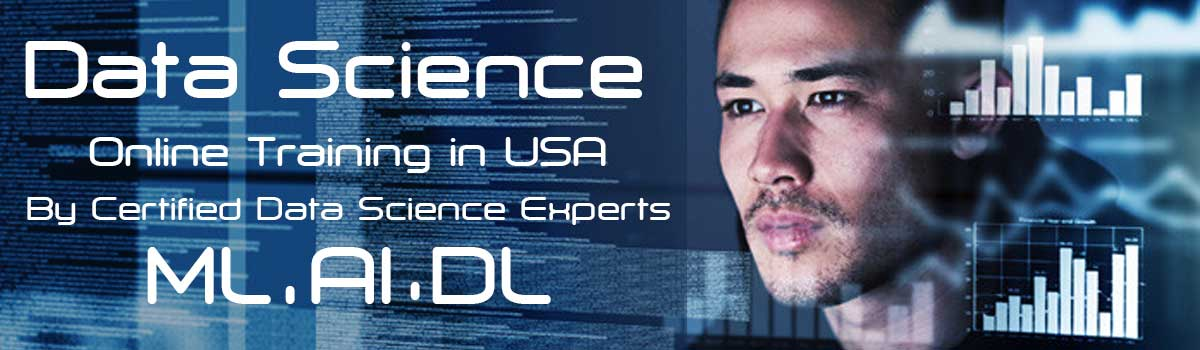# Data Science Online Training in USA

## Introduction to the Online Data Science Training in USA

We from TechSmartits providing Best Online Data Science Training in USA by Certified Data Science Experts with Data Analyst Job Placement Assistance. We are offering top Data Science Courses which include Machine Learning, Artificial Intelligence, Deep Learning all our training programs are handling by highly qualified certified experienced professionals, we assure that our students can experience hands on from day one on words.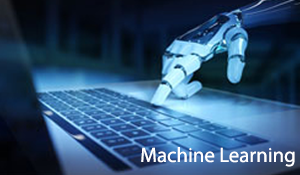#### Machine Learning

Machine learning is an application of artificial intelligence (AI) that provides systems the ability to automatically learn and improve from experience without being explicitly programmed.

#####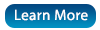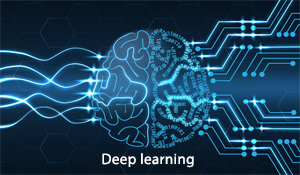#### Deep Learning

Deep learning is a subset of machine learning in artificial intelligence (AI) that has networks capable of learning unsupervised from data that is unstructured or unlabeled.

#####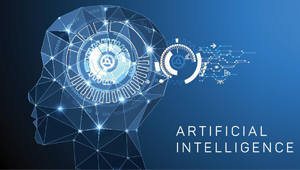#### Artificial intelligence

Artificial intelligence (AI) is the simulation of human intelligence processes by machines, especially computer systems. These processes include learning (the acquisition of information and rules for using the information).# Data Science Course Curriculum.

Section 1

• Introduction to Data Science
• Business Intelligence Vs Data Analysis
• Data Analysis Vs Data Scientist
• Data Scientist Roles.
• Different Disciplines of Data Science
• Machine Learning
• Natural Language Processing
• Deep Learning
• Artificial Intelligence
• When to use Machine Learning Models and Deep Learning Models.
• Applications of Machine Learning
• Why Machine Learning is the Future
• what are prerequisites for Data Science.
• Statistics
• R language essentials for Data Science
• Python essentials for Data Science
• [ Recorded Sessions of prerequisites will be given free of cost]
• different R language packages used for Data Science
• different Python modules used for Data Science
• Installing Python and Anaconda (Mac, Linux & Windows)
• Installing R and R Studio (Mac, Linux & Windows)

Section 2:  Data Preprocessing

• Why need to preprocess data
• Importing the Libraries
• Importing the Dataset
• python : overview of Object-oriented programming: classes & objects
• Missing Data
• Categorical Data
• Splitting the Dataset into the Training set and Test set
• Feature Scaling
• Data Preprocessing Template!

Section 3: Predictions using Regression

• Linear Vs Non Linear Regression
• Types of Linear Regressions
• what is slope and intercept.
• How to Derive Simple Linear Regression coefficients.
• Dataset + Business Problem Description
• Simple Linear Regression Intuition – Step 1
• Simple Linear Regression Intuition – Step 2
• Simple Linear Regression in Python – Step 1
• Simple Linear Regression in Python – Step 2
• Simple Linear Regression in Python – Step 3
• Simple Linear Regression in Python – Step 4
• Simple Linear Regression in R – Step 1
• Simple Linear Regression in R – Step 2
• Simple Linear Regression in R – Step 3
• Simple Linear Regression in R – Step 4

Section 4:  Multiple Linear Regression

• Dataset + Business Problem Description
• Multiple Linear Regression Intuition – Step 1
• Multiple Linear Regression Intuition – Step 2
• Multiple Linear Regression Intuition – Step 3
• Multiple Linear Regression Intuition – Step 4
• Prerequisites: What is the P-Value?
• Multiple Linear Regression Intuition – Step 5
• Multiple Linear Regression in Python – Step 1
• Multiple Linear Regression in Python – Step 2
• Multiple Linear Regression in Python – Step 3
• Multiple Linear Regression in Python – Backward Elimination – Preparation
• Multiple Linear Regression in Python – Backward Elimination – HOMEWORK !
• Multiple Linear Regression in Python – Backward Elimination – Homework Solution
• Multiple Linear Regression in Python – Automatic Backward Elimination
• Multiple Linear Regression in R – Step 1
• Multiple Linear Regression in R – Step 2
• Multiple Linear Regression in R – Step 3
• Multiple Linear Regression in R – Backward Elimination – HOMEWORK !
• Multiple Linear Regression in R – Backward Elimination – Homework Solution
• Multiple Linear Regression in R – Automatic Backward Elimination

Section 5:

• Polynomial Regression Intuition
• How to get the dataset
• Polynomial Regression in Python – Step 1
• Polynomial Regression in Python – Step 2
• Polynomial Regression in Python – Step 3
• Polynomial Regression in Python – Step 4
• Python Regression Template
• Polynomial Regression in R – Step 1
• Polynomial Regression in R – Step 2
• Polynomial Regression in R – Step 3
• Polynomial Regression in R – Step 4
• R Regression Template

Section 6: Support Vector Regression

• How to get the dataset
• SVR Intuition
• SVR in Python
• SVR in R

Section 7: Decision Tree Regression

• Decision Tree Regression Intuition
• How to get the dataset
• Decision Tree Regression in Python
• Decision Tree Regression in R

Section 8: Random Forest Regression

• Random Forest Regression Intuition
• How to get the dataset
• Random Forest Regression in Python
• Random Forest Regression in R

Section 9: Evaluating Regression Models.

• R-Squared Intuition
• Evaluating Regression Models Performance – Homework’s Final Part
• Interpreting Linear Regression Coefficients
• Developing Accuracy testing functions.

Section 10: Classification Algorithms

• What is a classification model.
• different types of classification models
• when to use what type of model
• how to measure accuracy of a classification model

Section 11 : Logistic Regression

• Logistic Regression Intuition
• How to get the dataset
• Logistic Regression in Python – Step 1
• Logistic Regression in Python – Step 2
• Logistic Regression in Python – Step 3
• Logistic Regression in Python – Step 4
• Logistic Regression in Python – Step 5
• Python Classification Template
• Logistic Regression in R – Step 1
• Logistic Regression in R – Step 2
• Logistic Regression in R – Step 3
• Logistic Regression in R – Step 4
• Logistic Regression in R – Step 5
• R Classification Template

Section 12: K-Nearest Neighbors [knn]

• K-Nearest Neighbor Intuition
• How to get the dataset
• K-NN in Python
• K-NN in R

Section 13: Support Vector Machine [svm]

• SVM Intuition
• How to get the dataset
• SVM in Python
• SVM in R

Section 14: Kernel SVM

• Kernel SVM Intuition
• Mapping to a higher dimension
• The Kernel Trick
• Types of Kernel Functions
• How to get the dataset
• Kernel SVM in Python
• Kernel SVM in R

Section 15: Naive Bayes Classifier

• Bayes Theorem
• Naive Bayes Intuition
• Naive Bayes Intuition (Challenge)
• Naive Bayes Intuition (Extras)
• How to get the dataset
• Naive Bayes in Python
• Naive Bayes in R

Section 16: Decision Tree Classifier

• Decision Tree Classification Intuition
• Entropy of Target variable
• Entropy of Input Variable on Target variable
• Information Gain
• How to get the dataset
• Decision Tree Classification in Python
• Decision Tree Classification in R

Section 17: Random Forest Classifier

• random Forest Classification Intuition
• how random forest works
• How to get the dataset
• Random Forest Classification in Python
• Random Forest Classification in R

Section 18: Evaluating classification models performance

• False Positives & False Negatives
• Confusion Matrix
• CAP Curve
• CAP Curve Analysis

Section 19: Clustering Algorithms

• What is a unsupervised learning.
• how to use unsupervised for business problems
• different Clustering models.

Section 20:  K-means Clustering

• K-Means Clustering Intuition
• K-Means Random Initialization Trap
• K-Means Selecting The Number Of Clusters
• How to get the dataset
• K-Means Clustering in Python
• K-Means Clustering in R

Section 21: Hierarchical Clustering

• Hierarchical Clustering Intuition
• Hierarchical Clustering How Dendrograms Work
• Hierarchical Clustering Using Dendrograms
• How to get the dataset
• HC in Python – Step 1
• HC in Python – Step 2
• HC in Python – Step 3
• HC in Python – Step 4
• HC in Python – Step 5
• HC in R – Step 1
• HC in R – Step 2
• HC in R – Step 3
• HC in R – Step 4
• HC in R – Step 5

Section 22: Association Rule Mining

• what are recommendation systems
• types of recommendation systems
• different ARM algorithms.
• how ARM used for recommendations.

Section 23: Apriori algorithm [one of arm]

• Apriori Intuition
• How to get the dataset
• Apriori in R – Step 1
• Apriori in R – Step 2
• Apriori in R – Step 3
• Apriori in Python – Step 1
• Apriori in Python – Step 2
• Apriori in Python – Step 3

Section 24: Eclat algorithm [ARM]

• Eclat Intuition
• How to get the dataset
• Eclat in R

Section 25: FPGrowth algorithm [ARM]

• FPGrowth Intuition
• problems with other ARM model.
• How to construct Growing tree.
• How to get the dataset
• FPGrowth in Python
• FPGrowth in R

Section 26: Reinforcement Learning

• What is Reinforcement Learning
• Models used in Reinforcement Learning

Section 27: Upper Confidence Bound [ part of Reinforcement Learning]

• The Multi-Armed Bandit Problem
• Upper Confidence Bound (UCB) Intuition
• How to get the dataset
• Upper Confidence Bound in Python – Step 1
• Upper Confidence Bound in Python – Step 2
• Upper Confidence Bound in Python – Step 3
• Upper Confidence Bound in Python – Step 4
• Upper Confidence Bound in R – Step 1
• Upper Confidence Bound in R – Step 2
• Upper Confidence Bound in R – Step 3
• Upper Confidence Bound in R – Step 4

Section 28: Thompson Sampling [part of Reinforcement Learning]

• Thompson Sampling Intuition
• Algorithm Comparison: UCB vs Thompson Sampling
• How to get the dataset
• Thompson Sampling in Python – Step 1
• Thompson Sampling in Python – Step 2
• Thompson Sampling in R – Step 1
• Thompson Sampling in R – Step 2

Section 29: Natural Language Processing

• what is nlp and its importance.
• what we can do with nlp
• Introduction to spam engines .
• Introduction to sentiment analyzers.
• word tokenization
• sentence tokenization
• parts of speech tagging
• lemmatization
• lemmatization
• removing stop words
• building word clouds
• feature extraction techniques and importance
• Word Existence feature
• Word proportion feature.
• TFIDF feature.
• NLP vs Machine Learning
• How to get the dataset
• Natural Language Processing in Python – Step 1
• Natural Language Processing in Python – Step 2
• Natural Language Processing in Python – Step 3
• Natural Language Processing in Python – Step 4
• Natural Language Processing in Python – Step 5
• Natural Language Processing in Python – Step 6
• Natural Language Processing in Python – Step 7
• Natural Language Processing in Python – Step 8
• Natural Language Processing in Python – Step 9
• Natural Language Processing in Python – Step 10
• Homework Challenge
• Natural Language Processing in R – Step 1
• Natural Language Processing in R – Step 2
• Natural Language Processing in R – Step 3
• Natural Language Processing in R – Step 4
• Natural Language Processing in R – Step 5
• Natural Language Processing in R – Step 6
• Natural Language Processing in R – Step 7
• Natural Language Processing in R – Step 8
• Natural Language Processing in R – Step 9
• Natural Language Processing in R – Step 10
• Homework Challenge

Section 30: Deep Learning

• problems of Machine Learning Models.
• Neural Networks
• How gradient descent algorithm works.
• what are Deep Neural Networks.

Section 31: Artificial Neural Networks [as part of Deep Learning]

• The Neuron
• The Activation Function
• How do Neural Networks work?
• How do Neural Networks learn?
• Back propagation
• How to get the dataset
• ANN in Python – Step 1 – Installing Theano, Tensor flow and Keras
• ANN in Python – Step 2
• ANN in Python – Step 3
• ANN in Python – Step 4
• ANN in Python – Step 5
• ANN in Python – Step 6
• ANN in Python – Step 7
• ANN in Python – Step 8
• ANN in Python – Step 9
• ANN in Python – Step 10
• ANN in R – Step 1
• ANN in R – Step 2
• ANN in R – Step 3
• ANN in R – Step 4 (Last step)

Section 32: Convolutional Neural Networks [as part of Deep Learning]

• What are convolutional neural networks?
• Step 1 – Convolution Operation
• Step 1(b) – ReLU Layer
• Step 2 – Pooling
• Step 3 – Flattening
• Step 4 – Full Connection
• Softmax & Cross-Entropy
• How to get the dataset
• CNN in Python – Step 1
• CNN in Python – Step 2
• CNN in Python – Step 3
• CNN in Python – Step 4
• CNN in Python – Step 5
• CNN in Python – Step 6
• CNN in Python – Step 7
• CNN in Python – Step 8
• CNN in Python – Step 9
• CNN in Python – Step 10
• CNN in R

Section 33: Reducing Dimensionality

• what is a dimension.
• why we should reduce dimensionality.
• different techniques to reduce

Section 34: Principal Compound Analysis

• PCA in Python – Step 1
• PCA in Python – Step 2
• PCA in Python – Step 3
• PCA in R – Step 1
• PCA in R – Step 2
• PCA in R – Step 3

Section 35: Linear Discriminate Analysis

• How to get the dataset
• LDA in Python
• LDA in R

Section 36: Kernal PCA

• How to get the dataset
• Kernel PCA in Python
• Kernel PCA in R

Section 37: Model Selection and Boosting

• How to select a model
• deferent techniques to select a model

Section 38: Model Selection

• How to get the dataset
• k-Fold Cross Validation in Python
• k-Fold Cross Validation in R
• Grid Search in Python – Step 1
• Grid Search in Python – Step 2
• Grid Search in R

Section 39: XGBoost

• how to get the dataset
• XGBoost in Python – Step 1
• XGBoost in Python – Step 2
• XGBoost in R